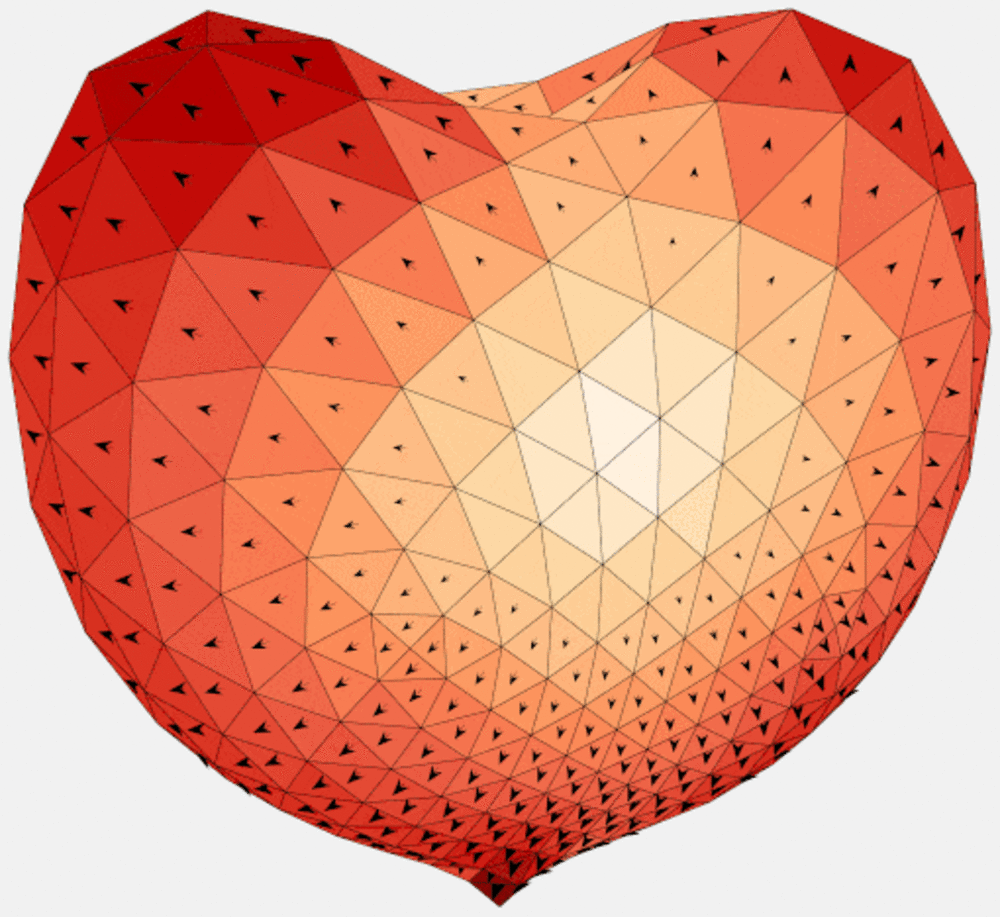# Method of MomentsMethod of moments is used to convert linear operator equations into linear algebraic equation systems by representing the solution in a suitable basis of expansion functions. The crucial step of identifying associated Green’s function makes this method ideal for open (radiating) problems since only the region containing sources is discretized.

Application of method of moments to integral equations leads to dense and relatively small (as compared the application to differential equations also known as finite element method) system matrix. Direct solvers are used to invert these matrices up to dimensions of several thousands. For bigger matrices, the efficient indirect solvers are available.

Conventionally, the inversion of a system matrix yields the contrast current flowing in the solution domain. This is, however, only one of many possibilities how to employ this method. Other techniques utilize the system matrix and its derivatives directly.

Figure: Surface current density (3rd characteristic mode) on a triangularized obstacle.

## Výzkumné skupiny### Computational Electromagnetics (CEM)

Výzkumné téma

Elektromagnetické pole

Výzkumný tým CEM se zaměřuje na výzkum teorie elektromagnetického pole. Mezi aktuálně rozvíjená témata patří výzkum malých elektrických zářičů a rozptylovačů a jejich optimalizace. Členové skupiny se již řadu let zabývají také klasickou teorií antén a šířením pole v umělých kompozitních materiálech.

Za stránku zodpovídá: Ing. Mgr. Radovan Suk